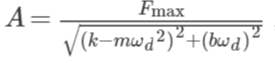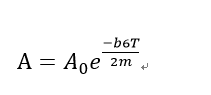# Damped Harmonic Oscillator and Resonance

## Homework Statement

On June 10, 2000, the Millennium Bridge, a new footbridge over the River Thames in London, England, was opened to the public. However, after only two days, it had to be closed to traffic for safety reasons. On the opening day, in fact, so many people were crossing it at the same time that unexpected sideways oscillations of the bridge were observed. Further investigations indicated that the oscillation was caused by lateral forces produced by the synchronization of steps taken by the pedestrians. Although the origin of this cadence synchronization was new to the engineers, its effect on the structure of the bridge was very well known. The combined forces exerted by the pedestrians as they were walking in synchronization had a frequency very close to the natural frequency of the bridge, and so resonance occurred

Consider an oscillating system of mass m and natural angular frequency ωn. When the system is subjected to a periodic external (driving) force, whose maximum value is Fmax and angular frequency is ωd, the amplitude of the driven oscillations is
where k is the force constant of the system and b is the damping constant.
We will use this simple model to study the oscillations of the Millennium Bridge.

Assume that, when we walk, in addition to a fluctuating vertical force, we exert a periodic lateral force of amplitude 25 N at a frequency of about 1 Hz. Given that the mass of the bridge is about 2000 kg per linear meter, how many people were walking along the 144-m-long central span of the bridge at one time, when an oscillation amplitude of 75 mm was observed in that section of the bridge? Take the damping constant to be such that the amplitude of the undriven oscillations would decay to 1/e of its original value in a time t=6T, where T is the period of the undriven, undamped system.

## Homework Equations## The Attempt at a Solution

I have difficulties in knowing what is resonance, so I start to solve the question by pluging each of the variables in the equation above.
First the oscillation amplitude is A which is 75x10-3m
Fmax is the external force to the system which is the bridge, therefore should be 25x no of people N in the bridge.
And ωd should be the angular frequency of the external periodical foce (not sure) which is 2pif =2pi rad/s
However, I can not determine what is the value of k and b. Thank you.

Sorry, can anyone help me?

TSny
Homework Helper
Gold Member
First the oscillation amplitude is A which is 75x10-3m
Fmax is the external force to the system which is the bridge, therefore should be 25x no of people N in the bridge.
And ωd should be the angular frequency of the external periodical foce (not sure) which is 2pif =2pi rad/s
OK.
However, I can not determine what is the value of k and b.
What can you deduce from the statement:
"Take the damping constant to be such that the amplitude of the undriven oscillations would decay to 1/e of its original value in a time t=6T, where T is the period of the undriven, undamped system"

So for the damping constant, the only way I can think of is the equation of damped oscillation which is
x=Ae-bt/2mcos(wt+fri) am I correct?

TSny
Homework Helper
Gold Member
Yes. You can see that the undriven amplitude decreases with time as ##\large{e^{-\frac{bt}{2m}}}##.

When deciding the amplitude, the term in cosine can be treated as the maximum which is one?

If this is correct, thenand A/A0 =1/e T is the period of the bridge system should be 1s (not sure)

TSny
Homework Helper
Gold Member
Yes. From this you can get a value of b/m.

now I get the frequency of the system and A, what about the value of k( the force constant of the system)?

TSny
Homework Helper
Gold Member
Do you need to know the value of k? Assume the system is being driven at or near resonance. How does the expression for A simplify near resonance?

Actually I have difficulties in figuring out what is meant by the force constant k. And what is shown by the equation above? Sorry for no pretty understand what is resonance completely.